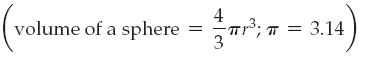×
Get Full Access to Introductory Chemistry - 5 Edition - Chapter 2 - Problem 104p
Get Full Access to Introductory Chemistry - 5 Edition - Chapter 2 - Problem 104p

×

# One of the particles in an atom is the proton. A proton has a radius of approximatelyISBN: 9780321910295 34

## Solution for problem 104P Chapter 2

Introductory Chemistry | 5th Edition

• Textbook Solutions
• 2901 Step-by-step solutions solved by professors and subject experts
• Get 24/7 help from StudySoup virtual teaching assistantsIntroductory Chemistry | 5th Edition

4 5 1 241 Reviews
13
2
Problem 104P

Problem 104P

One of the particles in an atom is the proton. A proton has a radius of approximately 1.0 × 10−13 cm and a mass of 1.7 × 10−24 g. Determine the density of a proton.Step-by-Step Solution:

Solution 104P

Step 1:

Density=Given that the mass of the proton is 1.7 × 10−24 g

The proton has a radius of 1.0 × 10−13 cm

Volume of the particle=4/3r3=4/3(1.0× 10−13)3

Step 2 of 2

##### ISBN: 9780321910295

The answer to “One of the particles in an atom is the proton. A proton has a radius of approximately 1.0 × 10?13 cm and a mass of 1.7 × 10?24 g. Determine the density of a proton.” is broken down into a number of easy to follow steps, and 35 words. Since the solution to 104P from 2 chapter was answered, more than 346 students have viewed the full step-by-step answer. This textbook survival guide was created for the textbook: Introductory Chemistry, edition: 5. This full solution covers the following key subjects: proton, mass, Density, determine, atom. This expansive textbook survival guide covers 19 chapters, and 2046 solutions. The full step-by-step solution to problem: 104P from chapter: 2 was answered by , our top Chemistry solution expert on 05/06/17, 06:45PM. Introductory Chemistry was written by and is associated to the ISBN: 9780321910295.

Unlock Textbook Solution